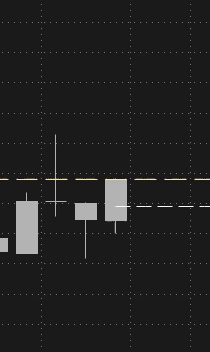# 25% and 75% added to this script

#### draw9300

##### Memberdef value = (high + low) / 2;
def value_fix = if isnan(value) then value_fix else value;

plot Fib50 = if isnan(close[-1]) then value_fix else double.nan;
Fib50.SetPaintingStrategy(PaintingStrategy.DASHES);
Fib50.SetDefaultColor(color.white);

As a candle expands the 75% and 25% lines could add more value along with the 50%. I don't know how to do add them

Solutiondef value = (high + low) / 2;
def value_fix = if isnan(value) then value_fix else value;

plot Fib50 = if isnan(close[-1]) then value_fix else double.nan;
Fib50.SetPaintingStrategy(PaintingStrategy.DASHES);
Fib50.SetDefaultColor(color.white);

As a candle expands the 75% and 25% lines could add more value along with the 50%. I don't know how to do add them

here is a code that draws 3 lines. can pick the % of the bar height to determine location.
if i am going to have multiple copies of the same code, i add a number to the variable name. per1, per2, per3.....
i could have used a script, but these are short enough that it wouldn't have gained much.

Ruby:
``````#
input per1 = 25;
#def x1 = (high + low) / 2;
def x1 =...``````def value = (high + low) / 2;
def value_fix = if isnan(value) then value_fix else value;

plot Fib50 = if isnan(close[-1]) then value_fix else double.nan;
Fib50.SetPaintingStrategy(PaintingStrategy.DASHES);
Fib50.SetDefaultColor(color.white);

As a candle expands the 75% and 25% lines could add more value along with the 50%. I don't know how to do add them

here is a code that draws 3 lines. can pick the % of the bar height to determine location.
if i am going to have multiple copies of the same code, i add a number to the variable name. per1, per2, per3.....
i could have used a script, but these are short enough that it wouldn't have gained much.

Ruby:
``````#
input per1 = 25;
#def x1 = (high + low) / 2;
def x1 = ((high - low) * (per1/100)) + low;
def x1_fix = if isnan(x1) then x1_fix else x1;
plot line1 = if isnan(close[-1]) then x1_fix else double.nan;
#line1.SetPaintingStrategy(PaintingStrategy.DASHES);
line1.SetStyle(Curve.short_DASH);
line1.SetDefaultColor(color.white);

input per2 = 50;
def x2 = ((high - low) * (per2/100)) + low;
def x2_fix = if isnan(x2) then x2_fix else x2;
plot line2 = if isnan(close[-1]) then x2_fix else double.nan;
line2.SetPaintingStrategy(PaintingStrategy.DASHES);
line2.SetDefaultColor(color.white);

input per3 = 75;
def x3 = ((high - low) * (per3/100)) + low;
def x3_fix = if isnan(x1) then x3_fix else x3;
plot line3 = if isnan(close[-1]) then x3_fix else double.nan;
#line3.SetPaintingStrategy(PaintingStrategy.DASHES);
line3.SetStyle(Curve.short_DASH);
line3.SetDefaultColor(color.white);

#``````

•draw9300

### Not the exact question you're looking for?

87k+ Posts
115 Online## The Market Trading Game Changer

Join 2,500+ subscribers inside the useThinkScript VIP Membership Club
• Exclusive indicators
• Proven strategies & setups
• Private Discord community
• Exclusive members-only content
• 1 full year of unlimited support

What is useThinkScript?

useThinkScript is the #1 community of stock market investors using indicators and other tools to power their trading strategies. Traders of all skill levels use our forums to learn about scripting and indicators, help each other, and discover new ways to gain an edge in the markets.

How do I get started?

We get it. Our forum can be intimidating, if not overwhelming. With thousands of topics, tens of thousands of posts, our community has created an incredibly deep knowledge base for stock traders. No one can ever exhaust every resource provided on our site.

If you are new, or just looking for guidance, here are some helpful links to get you started.

What are the benefits of VIP Membership?